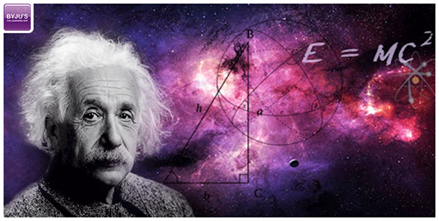# Physical LawsPhysical law is a scientific law that is a theoretical statement which is “inferred from particular facts, applicable to a defined group or class of phenomena, and expressible by the statement that a particular phenomenon always occurs if certain conditions are present.” (“Law of Nature” Oxford English Dictionary (3rd ed.). Oxford University Press. September 2005)

To explain it simply, when experiments were repeated again and again and observations were taken for a time period of years, the laws that survived this rigorous testing were considered universally as facts by scientists. Such laws are known as Physical laws. What science aims to do is to try and explain our surrounding ecosystem in terms of these laws.

To be called a Physical Law, a law must have these general properties:

• The law should be true inside the system it’s supposed to function in. Any new observations should not contradict the ones recorded in the past.
• The law should be applicable throughout the universe. Universally applicable.
• A single mathematical equation should be able to express the law. Simplicity is key.
• There should be no effect of any external or internal factors on it. In other words, it should be absolute.
• Stability is necessary. No change should have been since they were discovered.
• The observations should prove that everything in our universe should obey these laws. Or in other sense, they must be omnipotent.
• Should follow the conservation of quantity.
• The symmetry of space and time are expressed

Philosophiae Naturalis Principia Mathematica by Isaac Newton tells us the theories of classical mechanics and the theory of relativity by Einstein. Some of the other laws are the laws of thermodynamics and Boyle’s law of gas.

Whereas most people will simply give you a list of the laws, I’m not most people. We’ll take a look at the laws from their point of origin or wherever they were derived or conceptualized from. So put on seatbelts as things are gonna get interesting from here on!

Laws derived from Definitions

Few laws of science are derived from math definitions, for instance, the uncertainty principle, the principle of stationary action or causality. These laws are not mathematical as they are empirical and just explain what we perceive from our five senses.

Laws due to Mathematical Symmetries

The mathematical symmetries that are found in our nature, for instance, the rotational symmetry of space-time is reflected in the Lorentz transformation, the homogeneity of space is reflected in the conservation laws and the uniqueness of electrons is represented in the Pauli exclusion principle. Sometimes evidence appears in repeated experiments proving that the law is invalid or has loopholes, although it is highly unlikely that the physical laws would change.

Laws derived from Approximations

Sometimes general laws are modified or changed to form some of our physical laws. For instance, Special reactivity under low-speed approximations is Newtonian dynamics. General relativity in a low mass approximation is Newtonian Gravitation, when a situation that factors in large distances comes into play Quantum Electrodynamics is approximated into Coulomb’s law.

Laws derived from Symmetry Principles

Spacetime and such other symmetries result in mathematical consequences which are some approximated to create fundamental physical laws. For instance, when the symmetry of times shifts the conservation of energy is born as a consequence. The symmetry of space gives birth to the conservation of momentum.